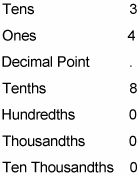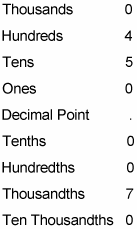##### Statistics: 1001 Practice Problems For Dummies (+ Free Online Practice)When you work with decimal numbers, you need to understand the difference between trailing zeros and leading zeros, as well as how they affect the value of the number.

You probably know that you can attach zeros to the beginning of a whole number without changing its value. For example, these three numbers are all equal in value:

27 027 0,000,027

The reason for this becomes clear when you know about place value of whole numbers. The following example attaches leading zeros to the value 27.As you can see, 0,000,027 simply means 0 + 0 + 0 + 0 + 0 + 20 + 7. No matter how many zeros you add on to the beginning of the number 27, it doesn’t change.

Zeros attached to the beginning of a number in this way are called leading zeros.

In decimals, this idea of zeros that don’t add value to a number can be extended to trailing zeros. A trailing zero is any zero that appears to the right of both the decimal point and every digit other than zero.

For example:

34.8 34.80 34.8000

All three of these numbers are the same. The reason becomes clear when you understand how place value works in decimals:In this example, 34.8000 means 30 + 4 + 8/10 + 0 + 0 + 0. You can attach or remove as many trailing zeros as you want to without changing the value of a number.

When you understand trailing zeros, you can see that every whole number can be changed to a decimal easily. Just attach a decimal point and a 0 to the end of it. For example:

4 = 4.0

20 = 20.0

971 = 971.0

Make sure that you don’t attach or remove any nonleading or nontrailing zeros, because doing this changes the value of the decimal.

For example, look at this number:

0450.0070

In this number, you can remove the leading and trailing zeros without changing the value, as follows:

450.007

The remaining zeros, however, need to stay where they are as placeholders between the decimal point and digits other than zero, as shown below.